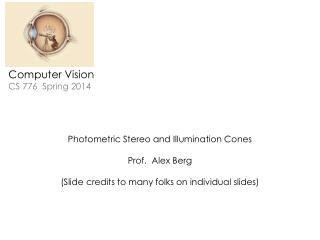# Computer Vision CS 776 Spring 2014 - PowerPoint PPT PresentationDownload PresentationComputer Vision CS 776 Spring 2014

Computer Vision CS 776 Spring 2014Download Presentation## Computer Vision CS 776 Spring 2014

- - - - - - - - - - - - - - - - - - - - - - - - - - - E N D - - - - - - - - - - - - - - - - - - - - - - - - - - -
##### Presentation Transcript

1. Computer VisionCS 776 Spring 2014 Photometric Stereo and Illumination Cones Prof. Alex Berg (Slide credits to many folks on individual slides)

2. Photometric Stereo and Illumination Cones flickr.com/photos/38102718@N05/3800198895/

3. Photometric stereo (shape from shading) • Can we reconstruct the shape of an object based on shading cues? Luca dellaRobbia,Cantoria, 1438 Slide by Svetlana Lazebnik

4. S2 S1 ??? Photometric stereo • Assume: • A Lambertian object • A local shading model (each point on a surface receives light only from sources visible at that point) • A set of known light source directions • A set of pictures of an object, obtained in exactly the same camera/object configuration but using different sources • Orthographic projection • Goal: reconstruct object shape and albedo Sn F&P 2nd ed., sec. 2.2.4

5. Surface model: Monge patch F&P 2nd ed., sec. 2.2.4

6. Image model • Known: source vectors Sjand pixel values Ij(x,y) • Unknown:normal N(x,y) and albedo ρ(x,y) • Assume that the response function of the camera is a linear scaling by a factor of k • Lambert’s law: F&P 2nd ed., sec. 2.2.4

7. (n × 1) (n × 3) (3× 1) known known unknown Least squares problem • Obtain least-squares solution for g(x,y) (which we defined asN(x,y) (x,y)) • Since N(x,y) is the unit normal, (x,y) is given by the magnitude of g(x,y) • Finally, N(x,y) = g(x,y) / (x,y) • For each pixel, set up a linear system: F&P 2nd ed., sec. 2.2.4

8. Example Recovered albedo Recovered normal field F&P 2nd ed., sec. 2.2.4

9. Recall the surface is written as This means the normal has the form: If we write the estimated vector gas Then we obtain values for the partial derivatives of the surface: Recovering a surface from normals F&P 2nd ed., sec. 2.2.4

10. Integrability: for the surface f to exist, the mixed second partial derivatives must be equal: We can now recover the surface height at any point by integration along some path, e.g. Recovering a surface from normals (for robustness, can take integrals over many different paths and average the results) (in practice, they should at least be similar) F&P 2nd ed., sec. 2.2.4

11. Surface recovered by integration F&P 2nd ed., sec. 2.2.4

12. More reading & thought problems (cone of images – great “old timey” figures) What is the set of images of an object under all possible lighting conditions? P. Belhumeur & D. Kriegman CVPR 1996 (implementing this one is the extra credit) Recovering High Dynamic Range Radiance Maps from Photographs. Paul E. Debevec and Jitendra Malik. SIGGRAPH 1997 (people can perceive reflectance) Surface reflectance estimation and natural illumination statistics. R.O. Dror, E.H. Adelson, and A.S. Willsky. Workshop on Statistical and Computational Theories of Vision 2001 gelsight.com